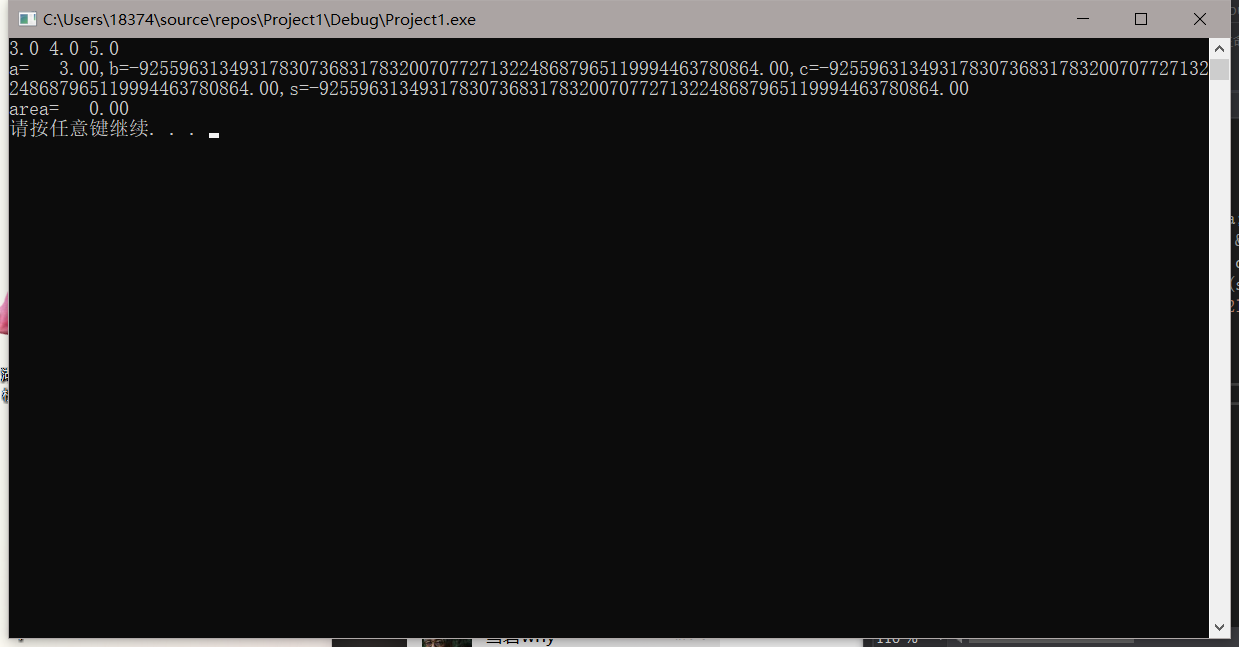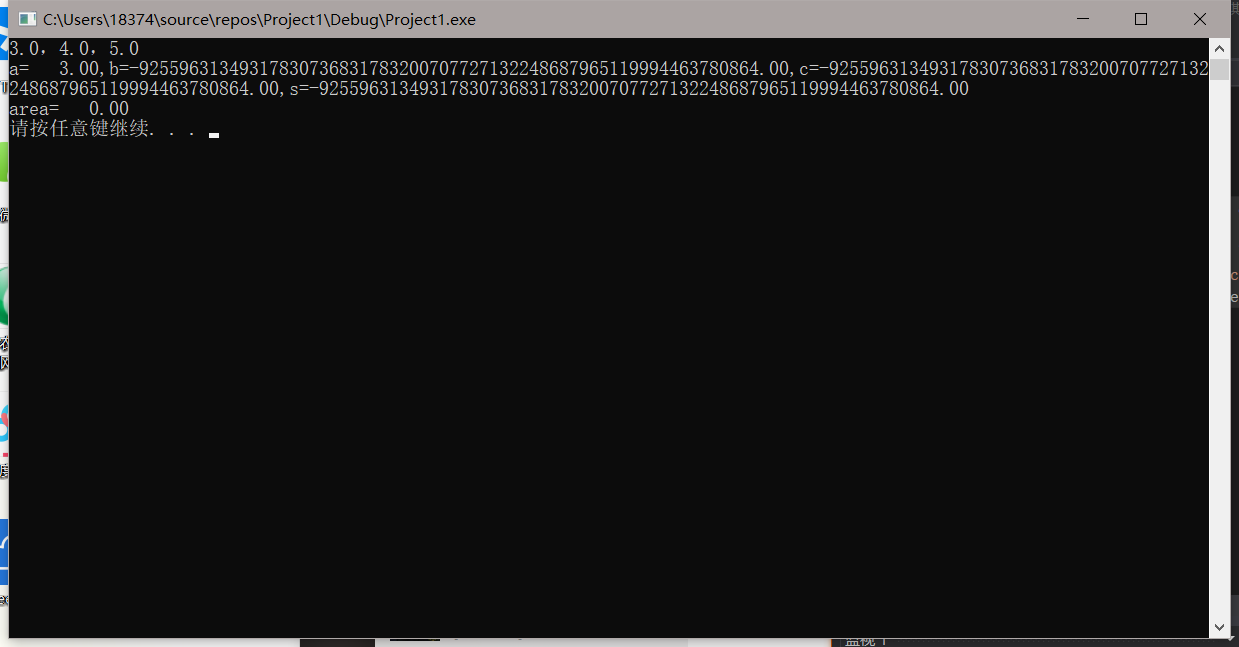``````#include<stdio.h>
#include<math.h>
void main()
{
double a, b, c, s, area;
scanf_s("%lf,%lf,%lf", &a, &b, &c);
s = 1.0 / 2 * (a + b + c);
area = sqrt(s*(s - a)*(s - b)*(s - c));
printf("a=%7.2lf,b=%7.2lf,c=%7.2lf,s=%7.2lf\n", a, b, c, s);
printf("area=%7.2lf\n", area);
system("pause");
}

``````4个回答

%7.2lf中的7是数据长度为七个字符的意思，若数据长度不够的话，左补空格；.2是表示对实数小数点后保留两位的意思。

%7.2lf 输出宽度为7，保留两位小数的精度

%7.2f 一般这么写，就是打印出浮点数总长度占7个字符的位置，其中保留两位小数1.问题：IP地址判断 描述 在基于Internet的程序中，我们常常需要判断一个IP字符串的合法性。合法的IP是这样的形式： A.B.C.D 其中A、B、C、D均为位于[0, 255]中的整数。为了简单起见，我们规定这四个整数中不会有前导零存在，如001这种情况。现在，请你来完成这个判断程序吧 输入 输入由多行组成，每行是一个字符串，输入由“End of file”结束。字符串长度最大为30，且不含空格和不可见字符。 输出 对于每一个输入，单独输出一行。如果该字符串是合法的IP，输出YES，否则， 输出NO 样例 输入 输出 202.115.32.24 YES a.b.c.d NO End of file 2.我自己写的一段代码 ``` #include <stdio.h> #include <string.h> int main() { char c; int i, d1, d2, d3, mid, zhi1, zhi2, zhi3, zhi4, oo，gd=0, m; while (1) { for (i = 0; i <= 35; i++) { c[i] = -1; } //初始化 i = 0; m = 0; while (c[m] != '\n') { c[i] = getchar(); m = i; i++; } oo = m - 1; if (strcmp(c, "End of file") == 0) break; else { for (i = 0; i <= 35; i++) //字符转成数字 { c[i] = c[i] - 48; } for (i = 0; i <= oo; i++) { if ((c[i] >= 10 || c[i] < 0) && c[i] != '.' - 48) gd++; } if (gd != 0 || (c[oo] < 0 || c[oo]>9)) { mid = 0; goto end; } //找到小数点。格式不符合规范就结束 for (d1 = 0; d1 <= 35; d1++) { if (c[d1] == '.' - 48) break; } for (d2 = d1 + 1; d2 <= 35; d2++) { if (c[d2] == '.' - 48) break; } for (d3 = d2 + 1; d3 <= 35; d3++) { if (c[d3] == '.' - 48) break; } if (d1 == 36 || d2 == 36 || d3 == 36 || d1 == 0 || d2 - d1 == 1 || d3 - d2 == 1 || d1 >= 4 || d2 - d1 - 1 >= 4 || d3 - d2 - 1 >= 4 || oo - d3 >= 4) { mid = 0; goto end; } //下面判断输入的值是否符合规范 //第一段 if (d1 == 3) { if (c == 0 || (c == 0 && c == 0)) { mid = 0; goto end; } else zhi1 = c * 100 + c * 10 + c; } else if (d1 == 2) { if (c == 0) { mid = 0; goto end; } else zhi1 = c * 10 + c; } else if (d1 == 1) { zhi1 = c; } //第二段 if (d2 - d1 == 4) { if (c[d1 + 1] == 0 || (c[d1 + 2] == 0 && c[d1 + 1] == 0)) { mid = 0; goto end; } else zhi2 = c[d1 + 1] * 100 + c[d1 + 2] * 10 + c[d1 + 3]; } else if (d2 - d1 == 3) { if (c[d1 + 1] == 0) { mid = 0; goto end; } else zhi2 = c[d1 + 1] * 10 + c[d1 + 2]; } else if (d2 - d1 == 2) { zhi2 = c[d1 + 1]; } //第三段 if (d3 - d2 == 4) { if (c[d2 + 1] == 0 || (c[d2 + 1] == 0 && c[d2 + 2] == 0)) { mid = 0; goto end; } else zhi3 = c[d2 + 1] * 100 + c[d2 + 2] * 10 + c[d2 + 3]; } else if (d3 - d2 == 3) { if (c[d2 + 1] == 0) { mid = 0; goto end; } else zhi3 = c[d2 + 1] * 10 + c[d2 + 2]; } else if (d3 - d2 == 2) { zhi3 = c[d2 + 1]; } //第四段 if (oo - d3 == 3) { if (c[d3 + 1] == 0 || (c[d3 + 1] == 0 && c[d3 + 2] == 0)) { mid = 0; goto end; } else zhi4 = c[d3 + 1] * 100 + c[d3 + 2] * 10 + c[d3 + 3]; } else if (oo - d3 == 2) { if (c[d3 + 1] == 0) { mid = 0; goto end; } else zhi4 = c[d3 + 1] * 10 + c[d3 + 2]; } else if (oo - d3 == 1) { zhi4 = c[d3 + 1]; } if (zhi1 >= 0 && zhi2 >= 0 && zhi3 >= 0 && zhi4 >= 0 && zhi1 <= 255 && zhi2 <= 255 && zhi3 <= 255 && zhi4 <= 255) { mid = 1; } else { mid = 0; } end: if (mid == 0) printf("NO\n"); else printf("YES\n"); } } return 0; } ``` 我试过了各种能想到的情况都是符合题目要求的，但是oj只给我了10.5/30分，我不知道有什么情况没有考虑到，求大神指点^o^。 ------------------------------------------------------------------------------- 谢谢大家，解决问题了^o^，循环的过程里面有变量的值改变了，我忘了在循环开始的时候初始化。不过还是谢谢大家的想法，我学到了很多呢！
STM32C语言位操作符的知识

Catch the cow(POJ3278) 编译器上没问题, OJ上一直runtime error?
[原题网址](http://poj.org/problem?id=3278 "Catch the cow") 下面是我已经在编译器上通过的代码,但是OJ上始终会RE (使用的是广度优先搜索的方法) ``` #include <stdio.h> #include <stdlib.h> #define MAX_N 100000 int n, k, ans; int que[MAX_N+10]; int vis[MAX_N+10]; int head, tail; void bfs( int x); void enqueue ( int x, int time); int main(void) { scanf("%d %d", &n, &k); if( n>k) { ans = n-k; } else { bfs(n); } printf("%d\n", ans); return 0; } void bfs( int x) { enqueue(x, 0); vis[x] = 1; while(head<tail) { int i, nowx, nowtime; nowx = que[head]; nowtime = que[head]; head ++; if( nowx == k) { ans = nowtime; break; } for( i=1; i<=3; i++) { if( i==1 && vis[nowx-1]!=1 && nowx-1>=0 && nowx-1 <= MAX_N) { enqueue( nowx-1, nowtime+1); vis[nowx-1] = 1; } if( i==2 && vis[nowx+1]!=1 && nowx+1>=0 && nowx+1 <= MAX_N) { enqueue( nowx+1, nowtime+1); vis[nowx+1] = 1; } if( i==3 && vis[nowx*2]!=1 && nowx*2>=0 && nowx*2 <= MAX_N) { enqueue( nowx*2, nowtime+1); vis[nowx*2] = 1; } } } } void enqueue( int x, int time) { que[tail] = x; que[tail] = time; tail ++; } ``` 一开始查了之后说可能是什么栈空间不够,就尝试了一下动态分配空间 (萌新还没学指针,就在网上照猫画虎贴了进去), 但是数字只要大一点程序就无法输出结果 更改后的代码如下: ``` #include <stdio.h> #include <stdlib.h> #define MAX_N 100001 int n, k, ans; //int que[MAX_N+10]; /*之前的方案,但同样RE了,可能是空间不足(?),所以尝试如下动态分配的方法*/ //int vis[MAX_N+10]; int head, tail; void bfs( int x, int **que, int *vis); void enqueue ( int x, int time, int **que); int main(void) { int **que; int i, j; int *vis; que = (int**)malloc(sizeof(int*)*MAX_N); //为两个数组分配空间 for( i=0; i<MAX_N; i++) { que[i] = (int*)malloc(sizeof(int)*2); } vis = (int*)malloc(sizeof(int)*MAX_N); scanf("%d %d", &n, &k); if( n>k) { ans = n-k; } else { bfs(n, que, vis); //进入深搜 } printf("%d\n", ans); return 0; } void bfs( int x, int **que, int *vis) { enqueue(x, 0, que); vis[x] = 1; while(head<tail) { int i, nowx, nowtime; nowx = que[head]; //队列数据的取出 nowtime = que[head]; head ++; if( nowx == k) //结束条件 { ans = nowtime; break; } for( i=1; i<=3; i++) //对三种可能进行遍历 { if( i==1 && vis[nowx-1]!=1 && nowx-1>=0 && nowx-1 <= MAX_N) { enqueue( nowx-1, nowtime+1, que); vis[nowx-1] = 1; } if( i==2 && vis[nowx+1]!=1 && nowx+1>=0 && nowx+1 <= MAX_N) { enqueue( nowx+1, nowtime+1, que); vis[nowx+1] = 1; } if( i==3 && vis[nowx*2]!=1 && nowx*2>=0 && nowx*2 <= MAX_N) { enqueue( nowx*2, nowtime+1, que); vis[nowx*2] = 1; } } } } void enqueue( int x, int time, int **que) //队列数据的写入 { que[tail] = x; que[tail] = time; tail ++; } ``` 请教一下大佬们上面RE的原因到底是什么, 还有下面的动态分配有什么问题,感激不尽
【c-WinAPI】 程序运行崩溃（自己写的函数出错），求大神帮忙！！！

File类的一个奇怪现象
<pre name="code" class="java">import java.io.File; public class Test { public static void main(String[] args) { File a = new File("d:"); System.out.println(a.getAbsolutePath()); } }</pre> <br /><span style="font-size: medium">上面这段代码生成的程序, 放在d盘以外的其他盘, 输出的是 d:\ <br /><img src="http://dl.iteye.com/upload/attachment/504807/26ab59dd-d89f-3377-a2f3-acbaac4d6a00.png" /> <br /> <br />但是放在d盘的任一文件夹下, 输出就变成了程序所在的文件夹 <br /><img src="http://dl.iteye.com/upload/attachment/504803/d2c58017-6e96-3a00-87c9-eb34e9ed2705.png" /> <br /> <br />感觉像是JDK的BUG, 但我看了File的源码, 字符串的解析却不是在java里做的... 貌似是用JNI扔给了windows <br /> <br />大惑不解, 求教&nbsp;<img src="/images/smiles/icon_mad.gif"/> <br /></span><br/><strong>问题补充</strong><br/><div class="quote_title">IcyFenix 写道</div><div class="quote_div">…… <br />相对路径啊，只说明是D盘不够，要说明是D盘根目录 <br />就是改成下面这样 <br />File a = new File("d:/");&nbsp; </div> <br />那为什么程序在其他盘的时候不会被认为是相对路径呢<br/><strong>问题补充</strong><br/><div class="quote_title">IcyFenix 写道</div><div class="quote_div">放到其他盘也是相对路径啊，只不过相对路径正好是D盘根目录而已。</div> <br />相对路径不是相对于当前目录的路径麽, 怎么会相对到其他盘的根目录了呢...&nbsp;<img src="/images/smiles/icon_mad.gif"/> <br/><strong>问题补充</strong><br/><div class="quote_title">captmjc 写道</div><div class="quote_div">lz当年肯定没学过dos，dos下面运行一下如下命令 <br />E: <br />CD E:\1 <br />DIR D: <br />PAUSE <br />CLS <br />CD D:\1 <br />DIR D: <br />PAUSE <br /> <br /></div> <br />终于有人看懂我的问题了，果然是跟windows有关，兄台厉害&nbsp;<img src="/images/smiles/icon_idea.gif"/> <br />PS: 当年的确是没学过DOS，因为DOS风行当年我还在读幼儿园&nbsp;<img src="/images/smiles/icon_smile.gif"/>

（接上文《源码阅读(18)：Java中主要的Map结构——HashMap容器（中）》） 3.4.4、HashMap添加K-V键值对（红黑树方式） 上文我们介绍了在HashMap中table数组的某个索引位上，基于单向链表添加新的K-V键值对对象（HashMap.Node&lt;K, V&gt;类的实例），但是我们同时知道在某些的场景下，HashMap中table数据的某个索引位上，数据是按照红黑树
c++制作的植物大战僵尸，开源，一代二代结合游戏
此游戏全部由本人自己制作完成。游戏大部分的素材来源于原版游戏素材，少部分搜集于网络，以及自己制作。 此游戏为同人游戏而且仅供学习交流使用，任何人未经授权，不得对本游戏进行更改、盗用等，否则后果自负。 目前有六种僵尸和六种植物，植物和僵尸的动画都是本人做的。qq：2117610943 开源代码下载 提取码：3vzm 点击下载--&gt; 11月28日 新增四种植物 统一植物画风，全部修
Java学习的正确打开方式

Python——画一棵漂亮的樱花树（不同种樱花+玫瑰+圣诞树喔）

linux系列之常用运维命令整理笔录

Python 基础（一）：入门必备知识

Python十大装B语法
Python 是一种代表简单思想的语言，其语法相对简单，很容易上手。不过，如果就此小视 Python 语法的精妙和深邃，那就大错特错了。本文精心筛选了最能展现 Python 语法之精妙的十个知识点，并附上详细的实例代码。如能在实战中融会贯通、灵活使用，必将使代码更为精炼、高效，同时也会极大提升代码B格，使之看上去更老练，读起来更优雅。 1. for - else 什么？不是 if 和 else 才

2019年11月中国大陆编程语言排行榜
2019年11月2日，我统计了某招聘网站，获得有效程序员招聘数据9万条。针对招聘信息，提取编程语言关键字，并统计如下： 编程语言比例 rank pl_ percentage 1 java 33.62% 2 c/c++ 16.42% 3 c_sharp 12.82% 4 javascript 12.31% 5 python 7.93% 6 go 7.25% 7

Python 编程实用技巧
Python是一门很灵活的语言，也有很多实用的方法，有时候实现一个功能可以用多种方法实现，我这里总结了一些常用的方法，并会持续更新。
“狗屁不通文章生成器”登顶GitHub热榜，分分钟写出万字形式主义大作

"狗屁不通文章生成器"登顶GitHub热榜，分分钟写出万字形式主义大作
GitHub 被誉为全球最大的同性交友网站，……，陪伴我们已经走过 10+ 年时间，它托管了大量的软件代码，同时也承载了程序员无尽的欢乐。 万字申请，废话报告，魔幻形式主义大作怎么写？兄dei，狗屁不通文章生成器了解一下。这个富有灵魂的项目名吸引了众人的目光。项目仅仅诞生一周，便冲上了GitHub趋势榜榜首（Js中文网 -前端进阶资源教程）、是榜首哦

《程序人生》系列-这个程序员只用了20行代码就拿了冠军

Spring Security 实战干货：基于注解的接口角色访问控制
1. 前言 欢迎阅读 Spring Security 实战干货 系列文章 。在上一篇 基于配置的接口角色访问控制 我们讲解了如何通过 javaConfig 的方式配置接口的角色访问控制。其实还有一种更加灵活的配置方式 基于注解 。今天我们就来探讨一下。DEMO 获取方式在文末。 2. Spring Security 方法安全 Spring Security 基于注解的安全...
8年经验面试官详解 Java 面试秘诀

1.两种思维方式在求职面试中，经常会考察这种问题：北京有多少量特斯拉汽车？某胡同口的煎饼摊一年能卖出多少个煎饼？深圳有多少个产品经理？一辆公交车里能装下多少个乒乓球？一个正常成年人有多少根头发？这类估算问题，被称为费米问题，是以科学家费米命名的。为什么面试会问这种问题呢？这类问题能把两类人清楚地区分出来。一类是具有文科思维的人，擅长赞叹和模糊想象，它主要依靠的是人的第一反应和直觉，比如小孩...

so easy！ 10行代码写个"狗屁不通"文章生成器

MySQL数据库总结

20行Python代码爬取王者荣耀全英雄皮肤